# Test: Squares, Square Roots, Cubes, Cube Roots

## 20 Questions MCQ Test Quantitative Techniques for CLAT | Test: Squares, Square Roots, Cubes, Cube Roots

Description
Attempt Test: Squares, Square Roots, Cubes, Cube Roots | 20 questions in 30 minutes | Mock test for Quant preparation | Free important questions MCQ to study Quantitative Techniques for CLAT for Quant Exam | Download free PDF with solutions
QUESTION: 1

Solution:
QUESTION: 2

Solution:
QUESTION: 3

### The value of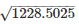is

Solution:
QUESTION: 4

The least number that must be added to 6072 to make it a perfect square is

Solution:
QUESTION: 5

The least number by which 12348 must be divided so as to have the quotient as a perfect square.

Solution:
QUESTION: 6

What must be subtracted from 4562 to get a perfect square

Solution:
QUESTION: 7

Find the greatest perfect square of 5 digits.

Solution:
QUESTION: 8

Find the least square number exactly divisible by 8, 9 and 10

Solution:
QUESTION: 9

What is the least number by which 13720 must be divided so that the quotient is a perfect cube

Solution:
QUESTION: 10

The value of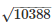given the value of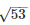= 7.28 is

Solution:
QUESTION: 11

The smallest number by which 980 must be multiplied so that the product is a perfect square

Solution:
QUESTION: 12

Which of the following is not correct

Solution:
QUESTION: 13

The value of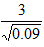Solution:= 3/0.3

= 10

QUESTION: 14

Of the numbers   0.16,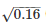, (0.16)2 and 0.016, the least is

Solution:
QUESTION: 15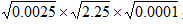equals

Solution:
QUESTION: 16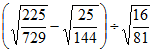equals

Solution:

terms after square root is

(15/27 - 5/12) / (4/9)

= 5/16

QUESTION: 17

The area of a square field is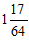cm2. The length of each side is

Solution:
QUESTION: 18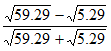equals

Solution:
QUESTION: 19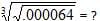Solution:
QUESTION: 20

What is the least number to be subtracted from 0.000326 to make it a perfect square.

Solution:Use Code STAYHOME200 and get INR 200 additional OFF Use Coupon Code